# Weight of a representation of a Lie algebra

(diff) ← Older revision | Latest revision (diff) | Newer revision → (diff)

in a vector spaceA linear mappingfrom the Lie algebrainto its field of definitionfor which there exists a non-zero vectorofsuch that for the representationone hasfor alland some integer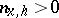(which in general depends onand). Here 1 denotes the identity transformation of. One also says in such a case thatis a weight of the-moduledefined by the representation. The set of all vectors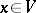which satisfy this condition, together with zero, forms a subspace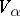, which is known as the weight subspace of the weight(or corresponding to). If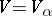, thenis said to be a weight space or weight module overof weight.

Ifis a finite-dimensional module overof weight, its contragredient module (cf. Contragredient representation)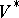is a weight module of weight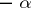; ifandare weight modules overof weightsand, respectively, then their tensor product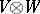is a weight module of weight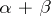. Ifis a nilpotent Lie algebra, a weight subspaceof weightinis an-submodule of the-module. If, in addition,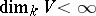andis a splitting Lie algebra of linear transformations of the module, thencan be decomposed into a direct sum of a finite number of weight subspaces of different weights:(the weight decomposition ofwith respect to). Ifis a nilpotent subalgebra of a finite-dimensional Lie algebra, considered as an-module with respect to the adjoint representation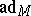of(cf. Adjoint representation of a Lie group), and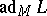is a splitting Lie algebra of linear transformations of, then the corresponding weight decomposition ofwith respect to,is called the Fitting decomposition ofwith respect to, the weightsare called the roots, while the spaces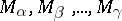are called the root subspaces ofwith respect to. If, in addition, one specifies the representationof the algebrain a finite-dimensional vector spacefor which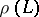is a splitting Lie algebra of linear transformations of, and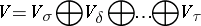is the corresponding weight decomposition ofwith respect to, thenif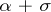is a weight ofwith respect to, and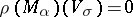otherwise. In particular, ifis a root, then, and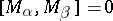otherwise. Ifis a field of characteristic zero, the weightsand the roots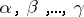are linear functions onwhich vanish on the commutator subalgebra of.

How to Cite This Entry:
Weight of a representation of a Lie algebra. Encyclopedia of Mathematics. URL: http://encyclopediaofmath.org/index.php?title=Weight_of_a_representation_of_a_Lie_algebra&oldid=49194
This article was adapted from an original article by V.L. Popov (originator), which appeared in Encyclopedia of Mathematics - ISBN 1402006098. See original article